# lime

Local interpretable model-agnostic explanations (LIME)

## Description

LIME explains a prediction of a machine learning model (classification or regression) for a query point by finding important predictors and fitting a simple interpretable model.

You can create a `lime` object for a machine learning model with a specified query point (`queryPoint`) and a specified number of important predictors (`numImportantPredictors`). The software generates a synthetic data set, and fits a simple interpretable model of important predictors that effectively explains the predictions for the synthetic data around the query point. The simple model can be a linear model (default) or decision tree model.

Use the fitted simple model to explain a prediction of the machine learning model locally, at the specified query point. Use the `plot` function to visualize the LIME results. Based on the local explanations, you can decide whether or not to trust the machine learning model.

Fit a new simple model for another query point by using the `fit` function.

## Creation

### Syntax

``results = lime(blackbox)``
``results = lime(blackbox,X)``
``results = lime(blackbox,'CustomSyntheticData',customSyntheticData)``
``results = lime(___,'QueryPoint',queryPoint,'NumImportantPredictors',numImportantPredictors)``
``results = lime(___,Name,Value)``

### Description

example

````results = lime(blackbox)` creates a `lime` object using a machine learning model object `blackbox` that contains predictor data. The `lime` function generates samples of a synthetic predictor data set and computes the predictions for the samples. To fit a simple model, use the `fit` function with `results`.```

example

````results = lime(blackbox,X)` creates a `lime` object using the predictor data in `X`.```
````results = lime(blackbox,'CustomSyntheticData',customSyntheticData)` creates a `lime` object using the pregenerated, custom synthetic predictor data set `customSyntheticData`. The `lime` function computes the predictions for the samples in `customSyntheticData`.```

example

````results = lime(___,'QueryPoint',queryPoint,'NumImportantPredictors',numImportantPredictors)` also finds the specified number of important predictors and fits a linear simple model for the query point `queryPoint`. You can specify `queryPoint` and `numImportantPredictors` in addition to any of the input argument combinations in the previous syntaxes.```
````results = lime(___,Name,Value)` specifies additional options using one or more name-value pair arguments. For example, `'SimpleModelType','tree'` specifies the type of simple model as a decision tree model.```

### Input Arguments

expand all

Machine learning model to be interpreted, specified as a function handle or a full or compact regression or classification model object.

Predictor data, specified as a numeric matrix or table. Each row of `X` corresponds to one observation, and each column corresponds to one variable.

`X` must be consistent with the predictor data that trained `blackbox`, stored in either `blackbox.X` or `blackbox.Variables`. The specified value must not contain a response variable.

• `X` must have the same data types as the predictor variables (for example, `trainX`) that trained `blackbox`. The variables that make up the columns of `X` must have the same number and order as in `trainX`.

• If you train `blackbox` using a numeric matrix, then `X` must be a numeric matrix.

• If you train `blackbox` using a table, then `X` must be a table. All predictor variables in `X` must have the same variable names and data types as in `trainX`.

• `lime` does not support a sparse matrix.

If `blackbox` is a compact machine learning model object or a function handle, you must provide `X` or `customSyntheticData`. If `blackbox` is a full machine learning model object and you specify this argument, then `lime` does not use the predictor data in `blackbox`. it uses the specified predictor data only.

Data Types: `single` | `double`

Pregenerated, custom synthetic predictor data set, specified as a numeric matrix or table.

If you provide a pregenerated data set, then `lime` uses the provided data set instead of generating a new synthetic predictor data set.

`customSyntheticData` must be consistent with the predictor data that trained `blackbox`, stored in either `blackbox.X` or `blackbox.Variables`. The specified value must not contain a response variable.

• `customSyntheticData` must have the same data types as the predictor variables (for example, `trainX`) that trained `blackbox`. The variables that make up the columns of `customSyntheticData` must have the same number and order as in `trainX`

• If you train `blackbox` using a numeric matrix, then `customSyntheticData` must be a numeric matrix.

• If you train `blackbox` using a table, then `customSyntheticData` must be a table. All predictor variables in `customSyntheticData` must have the same variable names and data types as in `trainX`.

• `lime` does not support a sparse matrix.

If `blackbox` is a compact machine learning model object or a function handle, you must provide `X` or `customSyntheticData`. If `blackbox` is a full machine learning model object and you specify this argument, then `lime` does not use the predictor data in `blackbox`; it uses the specified predictor data only.

Data Types: `single` | `double` | `table`

Query point at which `lime` explains a prediction, specified as a row vector of numeric values or a single-row table. `queryPoint` must have the same data type and number of columns as `X`, `customSyntheticData`, or the predictor data in `blackbox`.

If you specify `numImportantPredictors` and `queryPoint`, then the `lime` function fits a simple model when creating a `lime` object.

Example: `blackbox.X(1,:)` specifies the query point as the first observation of the predictor data in the full machine learning model `blackbox`.

Data Types: `single` | `double` | `table`

Number of important predictors to use in the simple model, specified as a positive integer scalar value.

• If `'SimpleModelType'` is `'linear'`, then the software selects the specified number of important predictors and fits a linear model of the selected predictors.

• If `'SimpleModelType'` is `'tree'`, then the software specifies the maximum number of decision splits (or branch nodes) as the number of important predictors so that the fitted decision tree uses at most the specified number of predictors.

If you specify `numImportantPredictors` and `queryPoint`, then the `lime` function fits a simple model when creating a `lime` object.

Data Types: `single` | `double`

Name-Value Pair Arguments

Specify optional comma-separated pairs of `Name,Value` arguments. `Name` is the argument name and `Value` is the corresponding value. `Name` must appear inside quotes. You can specify several name and value pair arguments in any order as `Name1,Value1,...,NameN,ValueN`.

Example: `lime(blackbox,'QueryPoint',q,'NumImportantPredictors',n,'SimpleModelType','tree')` specifies the query point as `q`, the number of important predictors to use for the simple model as `n`, and the type of simple model as a decision tree model. `lime` generates samples of a synthetic predictor data set, computes the predictions for the samples, and fits a decision tree model for the query point using at most the specified number of predictors.
Options for Synthetic Predictor Data

expand all

Locality of the synthetic data for data generation, specified as the comma-separated pair consisting of `'DataLocality'` and `'global'` or `'local'`.

• `'global'` — The software estimates distribution parameters using the whole predictor data set (`X` or the predictor data in `blackbox`). The software generates a synthetic predictor data set with the estimated parameters and uses the data set for simple model fitting of any query point.

• `'local'` — The software estimates the distribution parameters using the k-nearest neighbors of a query point, where k is the `'NumNeighbors'` value. The software generates a new synthetic predictor data set each time it fits a simple model for the specified query point.

For more details, see LIME.

Example: `'DataLocality','local'`

Data Types: `char` | `string`

Number of neighbors of the query point, specified as the comma-separated pair consisting of `'NumNeighbors'` and a positive integer scalar value. This argument is valid only when `'DataLocality'` is `'local'`.

If you specify a value larger than the number of observations in the predictor data set (`X` or the predictor data in `blackbox`), then `lime` uses all observations.

Example: `'NumNeighbors',2000`

Data Types: `single` | `double`

Number of samples to generate for the synthetic data set, specified as the comma-separated pair consisting of `'NumSyntheticData'` and a positive integer scalar value. This argument is valid only when `'DataLocality'` is `'local'`.

Example: `'NumSyntheticData',2500`

Data Types: `single` | `double`

Options for Simple Model

expand all

Kernel width of the squared exponential (or Gaussian) kernel function, specified as the comma-separated pair consisting of `'KernelWidth'` and a numeric scalar value.

The `lime` function computes distances between the query point and the samples in the synthetic predictor data set, and then converts the distances to weights by using the squared exponential kernel function. If you lower the `'KernelWidth'` value, then `lime` uses weights that are more focused on the samples near the query point. For details, see LIME.

Example: `'KernelWidth',0.5`

Data Types: `single` | `double`

Type of the simple model, specified as the comma-separated pair consisting of `'SimpleModelType'` and `'linear'` or `'tree'`.

Example: `'SimpleModelType','tree'`

Data Types: `char` | `string`

Options for Machine Learning Model

expand all

Categorical predictors list, specified as the comma-separated pair consisting of `'CategoricalPredictors'` and one of the values in this table.

ValueDescription
Vector of positive integersEach entry in the vector is an index value corresponding to the column of the predictor data that contains a categorical variable.
Logical vectorA `true` entry means that the corresponding column of the predictor data is a categorical variable.
Character matrixEach row of the matrix is the name of a predictor variable. The names must match the variable names of the predictor data in the form of a table. Pad the names with extra blanks so each row of the character matrix has the same length.
String array or cell array of character vectorsEach element in the array is the name of a predictor variable. The names must match the variable names of the predictor data in the form of a table.
`'all'`All predictors are categorical.

• If you specify `blackbox` as a function handle, then `lime` identifies categorical predictors from the predictor data `X` or `customSyntheticData`. If the predictor data is in a table, `lime` assumes that a variable is categorical if it is a logical vector, unordered categorical vector, character array, string array, or cell array of character vectors. If the predictor data is a matrix, `lime` assumes that all predictors are continuous. To identify any other predictors as categorical predictors, specify them by using the `'CategoricalPredictors'` name-value pair argument.

• If you specify `blackbox` as a regression or classification model object, then `lime` identifies categorical predictors by using the `CategoricalPredictors` property of the model object.

`lime` does not support an ordered categorical predictor.

Example: `'CategoricalPredictors','all'`

Data Types: `single` | `double` | `logical` | `char` | `string` | `cell`

Type of the machine learning model, specified as the comma-separated pair consisting of `'Type'` and `'regression` or `'classification'`.

You must specify this argument when you specify `blackbox` as a function handle. If you specify `blackbox` as a regression or classification model object, then `lime` determines the `'Type'` value depending on the model type.

Example: `'Type','classification'`

Data Types: `char` | `string`

Options for Computing Distances

expand all

Distance metric, specified as the comma-separated pair consisting of `'Distance'` and a character vector, string scalar, or function handle.

• If the predictor data includes only continuous variables, then `lime` supports these distance metrics.

ValueDescription
`'euclidean'`

Euclidean distance.

`'seuclidean'`

Standardized Euclidean distance. Each coordinate difference between observations is scaled by dividing by the corresponding element of the standard deviation, ```S = std(PD,'omitnan')```, where `PD` is the predictor data or synthetic predictor data. To specify different scaling, use the `'Scale'` name-value pair argument.

`'mahalanobis'`

Mahalanobis distance using the sample covariance of `PD`, ```C = cov(PD,'omitrows')```. To change the value of the covariance matrix, use the `'Cov'` name-value pair argument.

`'cityblock'`

City block distance.

`'minkowski'`

Minkowski distance. The default exponent is 2. To specify a different exponent, use the `'P'` name-value pair argument.

`'chebychev'`

Chebychev distance (maximum coordinate difference).

`'cosine'`

One minus the cosine of the included angle between points (treated as vectors).

`'correlation'`

One minus the sample correlation between points (treated as sequences of values).

`'spearman'`

One minus the sample Spearman's rank correlation between observations (treated as sequences of values).

`@distfun`

Custom distance function handle. A distance function has the form

```function D2 = distfun(ZI,ZJ) % calculation of distance ...```
where

• `ZI` is a `1`-by-`t` vector containing a single observation.

• `ZJ` is an `s`-by-`t` matrix containing multiple observations. `distfun` must accept a matrix `ZJ` with an arbitrary number of observations.

• `D2` is an `s`-by-`1` vector of distances, and `D2(k)` is the distance between observations `ZI` and `ZJ(k,:)`.

If your data is not sparse, you can generally compute distance more quickly by using a built-in distance metric instead of a function handle.

• If the predictor data includes both continuous and categorical variables, then `lime` supports these distance metrics.

ValueDescription
`'goodall3'`

Modified Goodall distance

`'ofd'`

Occurrence frequency distance

For definitions, see Distance Metrics.

The default value is `'euclidean'` if the predictor data includes only continuous variables, or `'goodall3'` if the predictor data includes both continuous and categorical variables.

Example: `'Distance','ofd'`

Data Types: `char` | `string` | `function_handle`

Covariance matrix for the Mahalanobis distance metric, specified as the comma-separated pair consisting of `'Cov'` and a K-by-K positive definite matrix, where K is the number of predictors.

This argument is valid only if `'Distance'` is `'mahalanobis'`.

The default `'Cov'` value is `cov(PD,'omitrows')`, where `PD` is the predictor data or synthetic predictor data. If you do not specify the `'Cov'` value, then the software uses different covariance matrices when computing the distances for both the predictor data and the synthetic predictor data.

Example: `'Cov',eye(3)`

Data Types: `single` | `double`

Exponent for the Minkowski distance metric, specified as the comma-separated pair consisting of `'P'` and a positive scalar.

This argument is valid only if `'Distance'` is `'minkowski'`.

Example: `'P',3`

Data Types: `single` | `double`

Scale parameter value for the standardized Euclidean distance metric, specified as the comma-separated pair consisting of `'Scale'` and a nonnegative numeric vector of length K, where K is the number of predictors.

This argument is valid only if `'Distance'` is `'seuclidean'`.

The default `'Scale'` value is `std(PD,'omitnan')`, where `PD` is the predictor data or synthetic predictor data. If you do not specify the `'Scale'` value, then the software uses different scale parameters when computing the distances for both the predictor data and the synthetic predictor data.

Example: ```'Scale',quantile(X,0.75) - quantile(X,0.25)```

Data Types: `single` | `double`

## Properties

expand all

### Specified Properties

You can specify the following properties when creating a `lime` object.

Machine learning model to be interpreted, specified as a regression or classification model object or a function handle.

The `blackbox` argument sets this property.

Categorical predictor indices, specified as a vector of positive integers. `CategoricalPredictors` contains index values corresponding to the columns of the predictor data that contain categorical predictors. If none of the predictors are categorical, then this property is empty (`[]`).

`lime` does not support an ordered categorical predictor.

If `'SimpleModelType'` is `'linear'`(default), then `lime` creates dummy variables for each identified categorical predictor. `lime` treats the category of the specified query point as a reference group and creates one less dummy variable than the number of categories. For more details, see Dummy Variables with Reference Group.

Data Types: `single` | `double`

Locality of the synthetic data for data generation, specified as `'global'` or `'local'`.

The `'DataLocality'` name-value pair argument sets this property.

Number of important predictors to use in the simple model (`SimpleModel`), specified as a positive integer scalar value.

The `numImportantPredictors` argument of `lime` or the `numImportantPredictors` argument of `fit` sets this property.

Data Types: `single` | `double`

Number of samples in the synthetic data set, specified as a positive integer scalar value.

Data Types: `single` | `double`

Query point at which `lime` explains a prediction using the simple model (`SimpleModel`), specified as a row vector of numeric values or single-row table.

The `queryPoint` argument of `lime` or the `queryPoint` argument of `fit` sets this property.

Data Types: `single` | `double` | `table`

Type of the machine learning model (`BlackboxModel`), specified as `'regression` or `'classification'`.

• If you specify `blackbox` as a regression or classification model object, then `lime` determines this property depending on the model type.

• If you specify `blackbox` using a function handle, then the `'Type'` name-value pair argument sets this property.

Predictor data, specified as a numeric matrix or table.

Each row of `X` corresponds to one observation, and each column corresponds to one variable.

• If you specify the `X` argument, then the argument sets this property.

• If you specify the `customSyntheticData` argument, then this property is empty.

• If you specify `blackbox` as a full machine learning model object and do not specify `X` or `customSyntheticData`, then this property value is the predictor data used to train `blackbox`.

Data Types: `single` | `double` | `table`

### Computed Properties

The software computes the following properties.

Prediction for the query point computed by the machine learning model (`BlackboxModel`), specified as a scalar.

Data Types: `single` | `double` | `categorical` | `logical` | `char` | `string` | `cell`

Predictions for synthetic predictor data computed by the machine learning model (`BlackboxModel`), specified as a vector.

Data Types: `single` | `double` | `categorical` | `logical` | `char` | `string` | `cell`

Important predictor indices, specified as a vector of positive integers. `ImportantPredictors` contains the index values corresponding to the columns of the predictors used in the simple model (`SimpleModel`).

Data Types: `single` | `double`

Simple model, specified as a `RegressionLinear`, `RegressionTree`, `ClassificationLinear`, or `ClassificationTree` model object. `lime` determines the type of simple model object depending on the type of the machine learning model (`Type`) and the type of the simple model (`'SimpleModelType'`).

Prediction for the query point computed by the simple model (`SimpleModel`), specified as a scalar.

If `SimpleModel` is `ClassificationLinear`, then the `SimpleModelFitted` value is 1 or –1.

• The `SimpleModelFitted` value is 1 if the prediction from the simple model is the same as `BlackboxFitted` (prediction from the machine learning model).

• The `SimpleModelFitted` value is –1 if the prediction from the simple model is different from `BlackboxFitted`. If the `BlackboxFitted` value is `A`, then the `plot` function displays the `SimpleModelFitted` value as `Not A`.

Data Types: `single` | `double` | `categorical` | `logical` | `char` | `string` | `cell`

Synthetic predictor data, specified as a numeric matrix or a table.

• If you specify the `customSyntheticData` input argument, then the argument sets this property.

• Otherwise, `lime` estimates distribution parameters from the predictor data `X` and generates a synthetic predictor data set.

Data Types: `single` | `double` | `table`

## Object Functions

 `fit` Fit simple model of local interpretable model-agnostic explanations (LIME) `plot` Plot results of local interpretable model-agnostic explanations (LIME)

## Examples

collapse all

Train a classification model and create a `lime` object that uses a decision tree simple model. When you create a `lime` object, specify a query point and the number of important predictors so that the software generates samples of a synthetic data set and fits a simple model for the query point with important predictors. Then display the estimated predictor importance in the simple model by using the object function `plot`.

Load the `CreditRating_Historical` data set. The data set contains customer IDs and their financial ratios, industry labels, and credit ratings.

`tbl = readtable('CreditRating_Historical.dat');`

Display the first three rows of the table.

`head(tbl,3)`
```ans=3×8 table ID WC_TA RE_TA EBIT_TA MVE_BVTD S_TA Industry Rating _____ _____ _____ _______ ________ _____ ________ ______ 62394 0.013 0.104 0.036 0.447 0.142 3 {'BB'} 48608 0.232 0.335 0.062 1.969 0.281 8 {'A' } 42444 0.311 0.367 0.074 1.935 0.366 1 {'A' } ```

Create a table of predictor variables by removing the columns of customer IDs and ratings from `tbl`.

`tblX = removevars(tbl,["ID","Rating"]);`

Train a blackbox model of credit ratings by using the `fitcecoc` function.

`blackbox = fitcecoc(tblX,tbl.Rating,'CategoricalPredictors','Industry');`

Create a `lime` object that explains the prediction for the last observation using a decision tree simple model. Specify `'NumImportantPredictors'` as six to find at most 6 important predictors. If you specify the `'QueryPoint'` and `'NumImportantPredictors'` values when you create a `lime` object, then the software generates samples of a synthetic data set and fits a simple interpretable model to the synthetic data set. Your results might vary from those shown because of randomness of `lime`. You can set a random seed by using `rng` for reproducibility.

`queryPoint = tblX(end,:)`
```queryPoint=1×6 table WC_TA RE_TA EBIT_TA MVE_BVTD S_TA Industry _____ _____ _______ ________ ____ ________ 0.239 0.463 0.065 2.924 0.34 2 ```
```results = lime(blackbox,'QueryPoint',queryPoint,'NumImportantPredictors',6, ... 'CategoricalPredictors','Industry','SimpleModelType','tree')```
```results = lime with properties: BlackboxModel: [1×1 ClassificationECOC] DataLocality: 'global' CategoricalPredictors: 6 Type: 'classification' X: [3932×6 table] QueryPoint: [1×6 table] NumImportantPredictors: 6 NumSyntheticData: 5000 SyntheticData: [5000×6 table] Fitted: {5000×1 cell} SimpleModel: [1×1 ClassificationTree] ImportantPredictors: [2 4] BlackboxFitted: {'AA'} SimpleModelFitted: {'AA'} ```

Plot the `lime` object `results` by using the object function `plot`. To display an existing underscore in any predictor name, change the `TickLabelInterpreter` value of the axes to `'none'`.

```f = plot(results); f.CurrentAxes.TickLabelInterpreter = 'none';```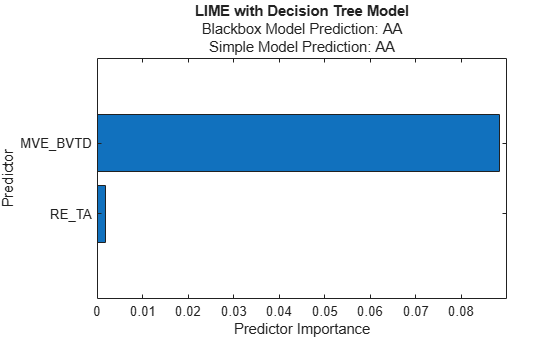The plot displays two predictions for the query point, which correspond to the BlackboxFitted property and the SimpleModelFitted property of `results`.

The horizontal bar graph shows the sorted predictor importance values. `lime` finds the financial ratio variables `EBIT_TA` and `WC_TA` as important predictors for the query point.

You can read the bar lengths by using data tips or Bar Properties. For example, you can find `Bar` objects by using the `findobj` function and add labels to the ends of the bars by using the `text` function.

```b = findobj(f,'Type','bar'); text(b.YEndPoints+0.001,b.XEndPoints,string(b.YData))```Alternatively, you can display the coefficient values in a table with the predictor variable names.

```imp = b.YData; flipud(array2table(imp', ... 'RowNames',f.CurrentAxes.YTickLabel,'VariableNames',{'Predictor Importance'}))```
```ans=2×1 table Predictor Importance ____________________ MVE_BVTD 0.088695 RE_TA 0.0018228 ```

Train a regression model and create a `lime` object that uses a linear simple model. When you create a `lime` object, if you do not specify a query point and the number of important predictors, then the software generates samples of a synthetic data set but does not fit a simple model. Use the object function `fit` to fit a simple model for a query point. Then display the coefficients of the fitted linear simple model by using the object function `plot`.

Load the `carbig` data set, which contains measurements of cars made in the 1970s and early 1980s..

`load carbig`

Create a table containing the predictor variables `Acceleration`, `Cylinders`, and so on, as well as the response variable `MPG`.

`tbl = table(Acceleration,Cylinders,Displacement,Horsepower,Model_Year,Weight,MPG);`

Removing missing values in a training set can help reduce memory consumption and speed up training for the `fitrkernel` function. Remove missing values in `tbl`.

`tbl = rmmissing(tbl);`

Create a table of predictor variables by removing the response variable from `tbl`.

`tblX = removevars(tbl,'MPG');`

Train a blackbox model of `MPG` by using the `fitrkernel` function, and create a `lime` object. Specify a predictor data set because `mdl` does not contain predictor data. Your results might vary from those shown because of randomness of `fitrkernel` and `lime`. You can set a random seed by using `rng` for reproducibility.

```mdl = fitrkernel(tblX,tbl.MPG,'CategoricalPredictors',[2 5]); results = lime(mdl,tblX,'CategoricalPredictors',[2 5])```
```results = lime with properties: BlackboxModel: [1×1 RegressionKernel] DataLocality: 'global' CategoricalPredictors: [2 5] Type: 'regression' X: [392×6 table] QueryPoint: [] NumImportantPredictors: [] NumSyntheticData: 5000 SyntheticData: [5000×6 table] Fitted: [5000×1 double] SimpleModel: [] ImportantPredictors: [] BlackboxFitted: [] SimpleModelFitted: [] ```

`results` contains the generated synthetic data set. The `SimpleModel` property is empty (`[]`).

Fit a linear simple model for the first observation in `tblX`. Specify the number of important predictors to find as 3.

`queryPoint = tblX(1,:)`
```queryPoint=1×6 table Acceleration Cylinders Displacement Horsepower Model_Year Weight ____________ _________ ____________ __________ __________ ______ 12 8 307 130 70 3504 ```
`results = fit(results,queryPoint,3);`

Plot the `lime` object `results` by using the object function `plot`. To display an existing underscore in any predictor name, change the `TickLabelInterpreter` value of the axes to `'none'`.

```f = plot(results); f.CurrentAxes.TickLabelInterpreter = 'none';```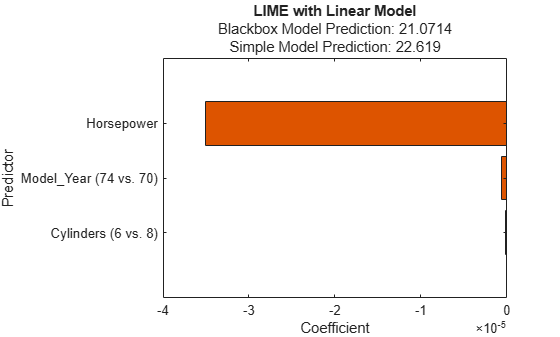The plot displays two predictions for the query point, which correspond to the BlackboxFitted property and the SimpleModelFitted property of `results`.

The horizontal bar graph shows the coefficient values of the simple model, sorted by their absolute values. LIME finds `Horsepower`, `Model_Year`, and `Cylinders` as important predictors for the query point.

Train a classification model and create a `lime` object that uses a decision tree simple model. Fit multiple models for multiple query points.

Load the `CreditRating_Historical` data set. The data set contains customer IDs and their financial ratios, industry labels, and credit ratings.

`tbl = readtable('CreditRating_Historical.dat');`

Create a table of predictor variables by removing the columns of customer IDs and ratings from `tbl`.

`tblX = removevars(tbl,["ID","Rating"]);`

Train a blackbox model of credit ratings by using the `fitcecoc` function.

`blackbox = fitcecoc(tblX,tbl.Rating,'CategoricalPredictors','Industry')`
```blackbox = ClassificationECOC PredictorNames: {'WC_TA' 'RE_TA' 'EBIT_TA' 'MVE_BVTD' 'S_TA' 'Industry'} ResponseName: 'Y' CategoricalPredictors: 6 ClassNames: {'A' 'AA' 'AAA' 'B' 'BB' 'BBB' 'CCC'} ScoreTransform: 'none' BinaryLearners: {21×1 cell} CodingName: 'onevsone' Properties, Methods ```

Create a `lime` object with the `blackbox` model. Your results might vary from those shown because of randomness of `lime`. You can set a random seed by using `rng` for reproducibility.

`results = lime(blackbox,'CategoricalPredictors','Industry');`

Find two query points whose true rating values are `AAA` and `B`, respectively.

```queryPoint(1,:) = tblX(find(strcmp(tbl.Rating,'AAA'),1),:); queryPoint(2,:) = tblX(find(strcmp(tbl.Rating,'B'),1),:)```
```queryPoint=2×6 table WC_TA RE_TA EBIT_TA MVE_BVTD S_TA Industry _____ _____ _______ ________ _____ ________ 0.121 0.413 0.057 3.647 0.466 12 0.019 0.009 0.042 0.257 0.119 1 ```

Fit a linear simple model for the first query point. Set the number of important predictors to 4.

`newresults1 = fit(results,queryPoint(1,:),4);`

Plot the LIME results `newresults1` for the first query point. To display an existing underscore in any predictor name, change the `TickLabelInterpreter` value of the axes to `'none'`.

```f1 = plot(newresults1); f1.CurrentAxes.TickLabelInterpreter = 'none';```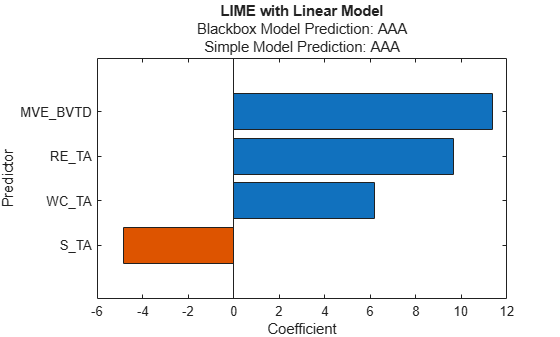Fit a linear decision tree model for the first query point.

```newresults2 = fit(results,queryPoint(1,:),6,'SimpleModelType','tree'); f2 = plot(newresults2); f2.CurrentAxes.TickLabelInterpreter = 'none';```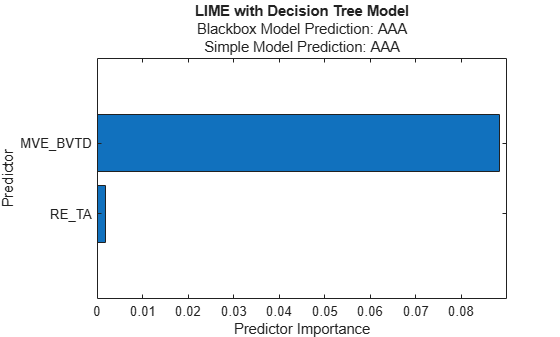The simple models in `newresults1` and `newresults2` both find `MVE_BVTD` and `RE_TA` as important predictors.

Fit a linear simple model for the second query point, and plot the LIME results for the second query point.

```newresults3 = fit(results,queryPoint(2,:),4); f3 = plot(newresults3); f3.CurrentAxes.TickLabelInterpreter = 'none';```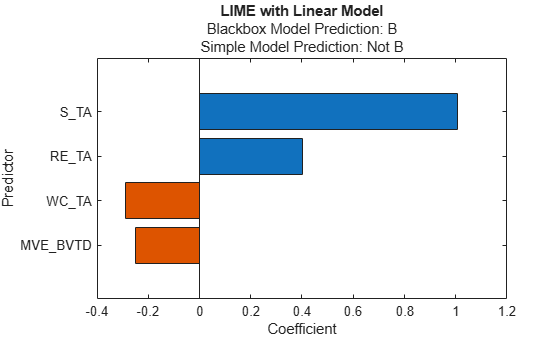The prediction from the `blackbox` model is `B`, but the prediction from the simple model is not `B`. When the two predictions are not the same, you can specify a smaller `'KernelWidth'` value. The software fits a simple model using weights that are more focused on the samples near the query point. If a query point is an outlier or is located near a decision boundary, then the two prediction values can be different, even if you specify a small `'KernelWidth'` value. In such a case, you can change other name-value pair arguments. For example, you can generate a local synthetic data set (specify `'DataLocality'` of `lime` as `'local'`) for the query point and increase the number of samples (`'NumSyntheticData'` of `lime` or `fit`) in the synthetic data set. You can also use a different distance metric (`'Distance'` of `lime` or `fit`).

Fit a linear simple model with a small `'KernelWidth'` value.

```newresults4 = fit(results,queryPoint(2,:),4,'KernelWidth',0.01); f4 = plot(newresults4); f4.CurrentAxes.TickLabelInterpreter = 'none';```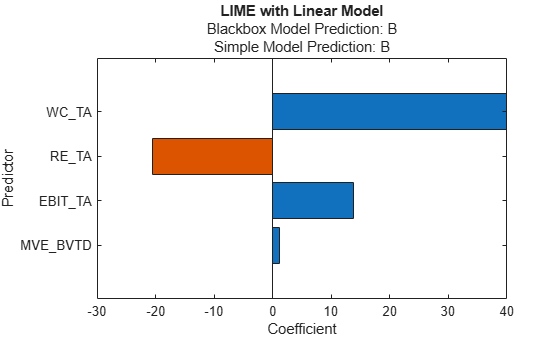The credit ratings for the first and second query points are `AAA` and `B`, respectively. The simple models in `newresults1` and `newresults4` both find `MVE_BVTD`, `RE_TA`, and `WC_TA` as important predictors. However, their coefficient values are different. The plots show that these predictors act differently depending on the credit ratings.

expand all

## Algorithms

expand all

 Ribeiro, Marco Tulio, S. Singh, and C. Guestrin. "'Why Should I Trust You?': Explaining the Predictions of Any Classifier." In Proceedings of the 22nd ACM SIGKDD International Conference on Knowledge Discovery and Data Mining, 1135–44. San Francisco California USA: ACM, 2016.

 Świrszcz, Grzegorz, Naoki Abe, and Aurélie C. Lozano. "Grouped Orthogonal Matching Pursuit for Variable Selection and Prediction." Advances in Neural Information Processing Systems (2009): 1150–58.

 Lozano, Aurélie C., Grzegorz Świrszcz, and Naoki Abe. "Group Orthogonal Matching Pursuit for Logistic Regression." Proceedings of the Fourteenth International Conference on Artificial Intelligence and Statistics (2011): 452–60.